##### Managerial Economics For Dummies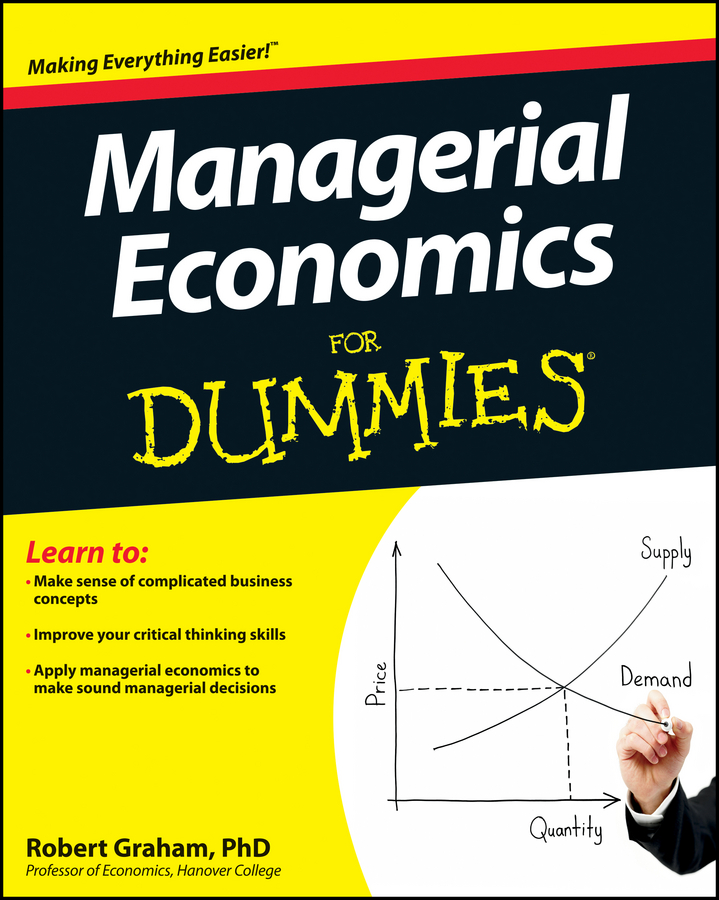Typically a new product or new production technique isn’t successful. Because of the high risk and likelihood of failure, you can often reduce research and development costs by simultaneously working on several similar or parallel ideas. Ongoing evaluation of costs and potential benefits of the parallel efforts enables you to determine at what point you should abandon an effort in order to reduce research and development costs.

Your pharmaceutical company is developing two different drugs for the same medical condition. Each drug’s development cost is influenced by a number of factors, such as potential side effects and other drug interactions. Because development costs are unknown, you develop best-case and worst-case scenarios that you assume are the same for both drugs.

Your best-case scenario for either drug is that the development cost equals \$20 million. Your worst-case scenario for either drug is that the development cost equals \$75 million. Because you’re unsure what the actual development cost will be for each drug, as a pessimist you assume a 30-percent chance for the best-case scenario and a 70-percent chance for the worst-case scenario.

Alternatively, you could be an optimist and use a higher probability for the best-case scenario, or you could base your probability on past experience.

The expected development cost, EDC, for each drug equals the cost of a scenario, Cbc for the best-case scenario and Cwc for the worst-case scenario, multiplied by the probability of that scenario occurring, Pbc and Pwc, or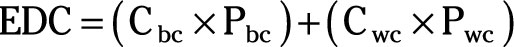You use the following steps to calculate the expected development cost for a drug:

1. First, substitute the values for Cbc, Pbc, Cwc, and Pwc.

2. For each scenario, multiply the cost by the probability.

For the best-case scenario, multiply \$20 million by 0.3, and for the worst-case scenario, multiply \$75 million by 0.7.

3. Add the resulting values for each scenario.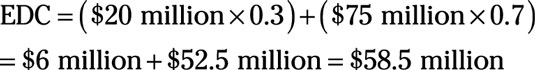The expected development cost equals \$58.5 million.

Starting your research by developing both drugs at the same time can lower your expected development cost because partway through the process, you can decide to abandon one drug because its development costs are too high.

If both drugs are developed in parallel, you have a 49-percent chance (0.7 × 0.7) that the development cost of each drug equals \$75 million. This is the probability of the worst-case scenario occurring for both drugs. You have a 9-percent chance (0.3 × 0.3) chance that the development cost of each drug equals \$20 million. This is your best-case scenario occurring for both drugs.

Finally, you have a 42-percent chance that one of the two drugs has the best case scenario — a \$20 million development cost. This is the situation where one drug’s development represents the best-case scenario and the other drug’s development represents the worst-case scenario (0.3 × 0.7 + 0.7 × 0.3).

Because you ultimately complete the development of only one drug — remember, both drugs are for the same condition — you’ll choose the cheapest drug to develop. Thus, you have a 51-percent chance — the 9-percent chance of best-case for both drugs, plus the 42-percent chance of best-case for one of the two drugs — that the development cost equals \$20 million.

Note how 51 percent is better than the 30 percent chance of the best-case scenario’s \$20 million development cost if you develop only one drug.

Now comes a critical step. If the actual development cost of each drug can be determined with certainty after \$C have been spent, and only the drug with the lowest development cost is developed after that point, the expected development cost equals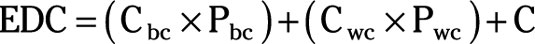In the example, you know that you can determine the actual development cost for each drug after spending \$8 million. (This value varies from situation to situation and must be determined in advance, usually by past experience.) Thus, your expected development cost of parallel efforts equals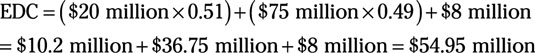In this situation, your parallel efforts have lowered the expected development cost by \$3.55 million from the initial \$58.5 million to \$54.95 million.